Worksheets and No Prep Teaching Resources
Math Worksheets
Measurement Worksheets

# 2nd Grade MeasurementWorksheets, Lessons, and Printables

Measuring Length
Easier: First Answer Given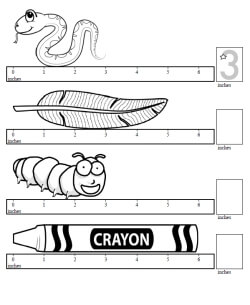Measure length in inches - first answer given Measure length in centimeters - first answer given

Measuring Length of ObjectsMeasure length in inches Measure length in centimeters

Sorting Length
Easier: First Answer and Last Answer Given (Preschool and Kindergarten)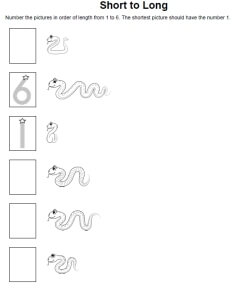Measure length in inches - 1 is shortest, 6 is longest (first and last answers given) Measure length in inches - 1 is longest, 6 is shortest (first and last answers given) Measure length in inches - mix (first and last answers given)

Sorting Length: First Answer Given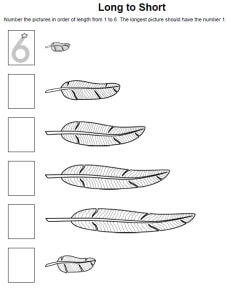Measure length in inches - 1 is shortest, 6 is longest (first answer given) Measure length in inches - 1 is longest, 6 is shortest (first answer given) Measure length in inches - mix (first answer given)

Sorting Length: One Answer Given (can be any number from 1 to 6)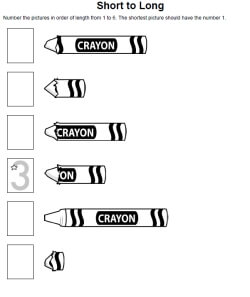Measure length in inches - 1 is shortest, 6 is longest (one answer given) Measure length in inches - 1 is longest, 6 is shortest (one answer given) Measure length in inches - mix (one answer given)

Length of Lines

Inches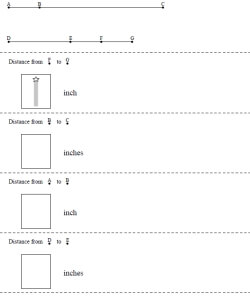Measure length in inches between points

Centimeters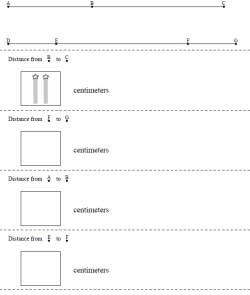Measure length in centimeters between points

Length of Boxes
Easier: Always the Same Direction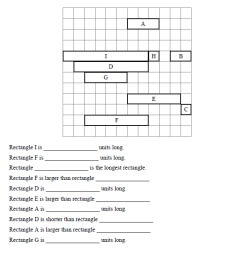Length: One large problem (4 to 6 rectangles) Length: One large problem (7 to 10 rectangles) Length: One large problem (11 to 15 rectangles)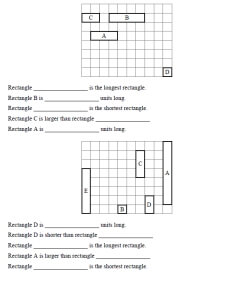Length: Two problems per page (4 to 6 rectangles) Length: Two problems per page (7 to 10 rectangles)

Different Directions on Same Problem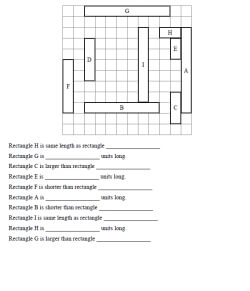Length: One large problem (4 to 6 rectangles) Length: One large problem (7 to 10 rectangles) Length: One large problem (11 to 15 rectangles)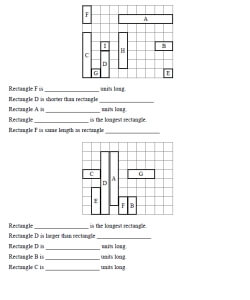Length: Two problems per page (4 to 6 rectangles) Length: Two problems per page (7 to 10 rectangles)

With Addition and Subtraction Questions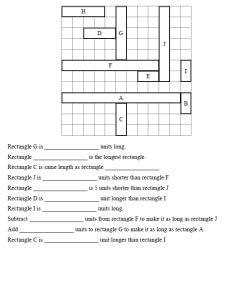Length with Addition and Subtraction Questions: One large problem (4 to 6 rectangles) Length with Addition and Subtraction Questions: One large problem (7 to 10 rectangles) Length with Addition and Subtraction Questions: One large problem (11 to 15 rectangles)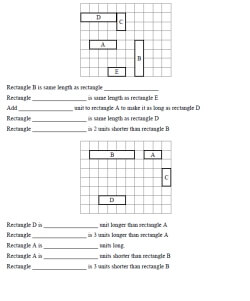Length with Addition and Subtraction Questions: Two problems per page (4 to 6 rectangles) Length with Addition and Subtraction Questions: Two problems per page (7 to 10 rectangles)

Measure Length of Line Segments

Length and Weight (Grades 1-3)
Length (black and white graphics)
Circle the object with the longest side
Number the objects in order from shortest to longest
Number the objects in order from longest to shortest
Estimate (inches and feet)
Estimate (centimeters and meters)
Weight (black and white graphics)
Circle the object that is heavier
Circle the object that is lighter
Number the objects in order from lightest to heaviest
Number the objects in order from heaviest to lightest
Estimate (ounces and pounds)
Estimate (grams and kilograms)
Review (black and white graphics)
Length and weight review (black and white graphics)

Length (color graphics)
Circle the object with the longest side
Number the objects in order from shortest to longest
Number the objects in order from longest to shortest
Estimate (inches and feet)
Estimate (centimeters and meters)
Weight (color graphics)
Circle the object that is heavier
Circle the object that is lighter
Number the objects in order from lightest to heaviest
Number the objects in order from heaviest to lightest
Estimate (ounces and pounds)
Estimate (grams and kilograms)
Review (color graphics)
Length and weight review (color graphics)

Make the Scale Balance
Easier: Balance is Equal - Add the Missing Blocks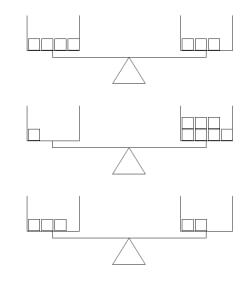Add the missing blocks Add the missing blocks and write the number of blocks on each side Add the missing blocks - one problem does not need any blocks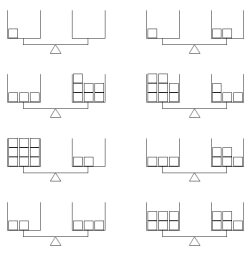More problems per page More problems per page - one problem does not need any blocks

One Side of Balance is Heavier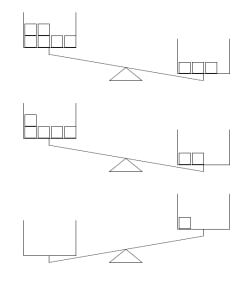Add the missing blocks Add the missing blocks and write the number of blocks on each side Add the missing blocks - one problem does not need any blocks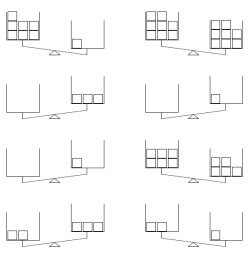More problems per page More problems per page - one problem does not need any blocks

Some Balances are Equal - Others have Heavier Sides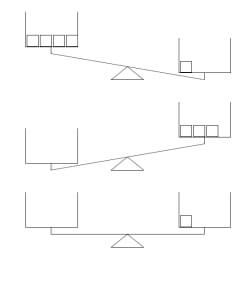Add the missing blocks Add the missing blocks and write the number of blocks on each side Add the missing blocks - one problem does not need any blocks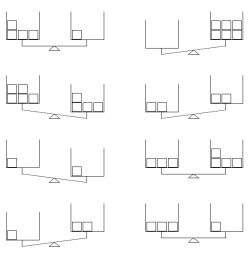More problems per page More problems per page - one problem does not need any blocks

Greater and Less Than: Make the Scale Balance
Easier: Maximum Sum of 19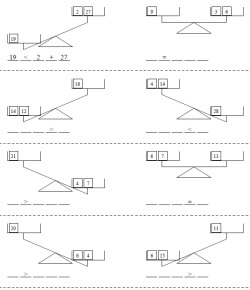Answer given for the first problem - the sign is given for all the problems Answer given for the first problem - the sign is given for the first four problems Answer given for the first problem No answers, but blank lines are shown for parts ___ ____ ____ ____ _____
Maximum Sum of 99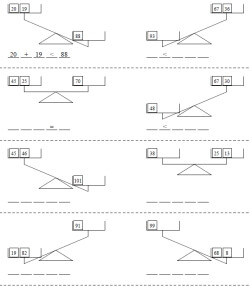Answer given for the first problem - the sign is given for all the problems Answer given for the first problem - the sign is given for the first four problems Answer given for the first problem No answers, but blank lines are shown for parts ___ ____ ____ ____ _____
Algebra Challenge - Maximum Sum of 19 - All balances are equal and there is one UNKNOWN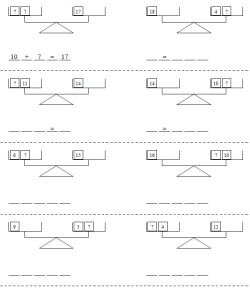Algebra Challenge: Answer given for the first problem - the sign is given for all the problems Algebra Challenge: Answer given for the first problem - the sign is given for the first four problems Algebra Challenge: Answer given for the first problem Algebra Challenge: No answers, but blank lines are shown for parts ___ ____ ____ ____ _____

Using a Ruler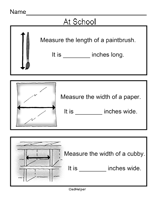I Can Measure It Myself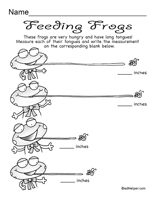Ruler Puzzles

Easiest: Measurement Math Center - Inches - Fewer Images on Cards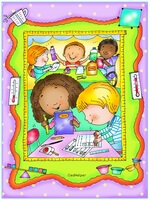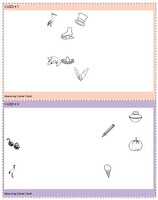Easiest: Measure the length between two pictures - Horizontal Measure Only (full page cards) (Grades 1-4) Easiest: Measure the length between two pictures - Horizontal Measure Only (half-page cards) (Grades 1-4) Easiest: Measure the length between two pictures - Vertical Measure Only (full page cards) (Grades 1-4) Easiest: Measure the length between two pictures - Vertical Measure Only (half-page cards) (Grades 1-4) Measure the length between two pictures - Horizontal or Vertical Measure (full page cards) (Grades 1-4) Measure the length between two pictures - Horizontal or Vertical Measure (half-page cards) (Grades 1-4) Measure the length between two pictures (full page cards) (Grades 1-4) Measure the length between two pictures (half-page cards) (Grades 1-4)

Measurement Math Center - InchesMeasure the length between two pictures (full page cards) (Grades 1-4) Measure the length between two pictures (half-page cards) (Grades 1-4)

Easiest: Measurement Math Center - Centimeters - Fewer Images on CardsEasiest: Measure the length between two pictures - Horizontal Measure Only (full page cards) (Grades 1-4) Easiest: Measure the length between two pictures - Horizontal Measure Only (half-page cards) (Grades 1-4) Easiest: Measure the length between two pictures - Vertical Measure Only (full page cards) (Grades 1-4) Easiest: Measure the length between two pictures - Vertical Measure Only (half-page cards) (Grades 1-4) Measure the length between two pictures - Horizontal or Vertical Measure (full page cards) (Grades 1-4) Measure the length between two pictures - Horizontal or Vertical Measure (half-page cards) (Grades 1-4) Measure the length between two pictures (full page cards) (Grades 1-4) Measure the length between two pictures (half-page cards) (Grades 1-4)

Measurement Math Center - CentimetersMeasure the length between two pictures (full page cards) (Grades 1-4) Measure the length between two pictures (half-page cards) (Grades 1-4)

Bulletin Board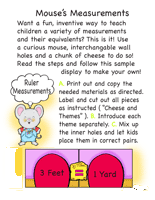Mouse's Measurements

Cooking Activities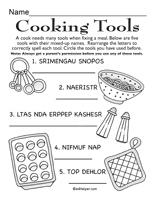Cooking Puzzles

Volume Capacity: Circle the Bottle with More or with Less Water
Easier: Bottle Sizes the Same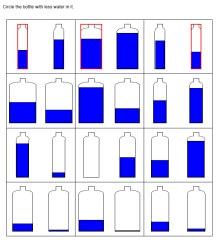Circle the Bottle with More (answers on first row are given) Circle the Bottle with Less (answers on first row are given) Each page will randomly pick either More or Less (answers on first row are given)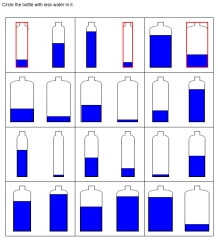Circle the Bottle with More Circle the Bottle with Less Each page will randomly pick either More or Less

More Difficult: Different Size Bottles in Problems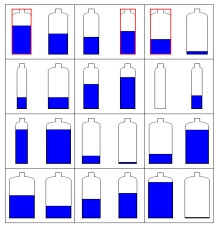Circle the Bottle with More (answers on first row are given) Circle the Bottle with Less (answers on first row are given) Each page will randomly pick either More or Less (answers on first row are given)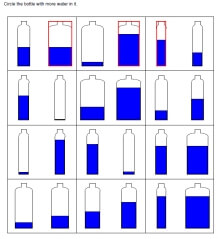Circle the Bottle with More Circle the Bottle with Less Each page will randomly pick either More or Less

Order Capacity: Order the objects from smallest capacity to greatest capacity
Order Capacity: Order the objects from greatest capacity to smallest capacity
Circle the container that holds more
Circle the container that holds less

Cups and Pints
Circle the set which holds more
Circle the set which holds less
Which holds more? (more difficult)
Which holds less? (more difficult)

Pints and Quarts
Circle the set which holds more
Circle the set which holds less
Which holds more? (more difficult)
Which holds less? (more difficult)

Cups and Quarts
Circle the set which holds more
Circle the set which holds less
Which holds more? (more difficult)
Which holds less? (more difficult)

Cups, Pints, and Quarts
Circle the set which holds more
Circle the set which holds less
Which holds more? (more difficult)
Which holds less? (more difficult)

Quarts and Gallons
Circle the set which holds more
Circle the set which holds less
Which holds more? (more difficult)
Which holds less? (more difficult)

Pints, Quarts, and Gallons
Circle the set which holds more
Circle the set which holds less
Which holds more? (more difficult)
Which holds less? (more difficult)

Mix
Cups and pints
Cups, pints, and quarts
Cups, pints, quarts, and gallons

Temperature
Is the temperature hot or cold in the picture?
Color the thermometer to show the temperature
Color the thermometer to show the temperature

Capacity Review
Capacity mixed review

Temperature: Circle the Warmer or Colder Temperature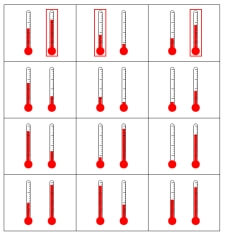Circle the warmer temperature (answers on first row are given) Circle the colder temperature (answers on first row are given) Each page will randomly pick either warmer or colder (answers on first row are given)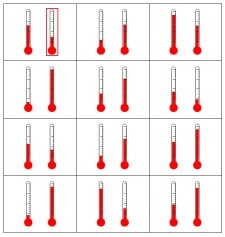Circle the warmer temperature Circle the colder temperature Each page will randomly pick either warmer or colder

Temperature: Write the Temperature and Color in the Temperature
Fahrenheit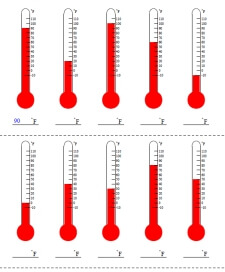Fahrenheit: Write the temperature - no negatives and tens only Fahrenheit: Write the temperature - tens only Fahrenheit: Write the temperature - no negatives Fahrenheit: Write the temperature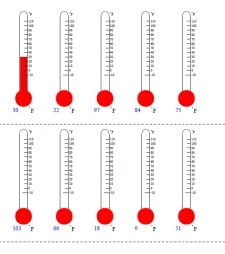Fahrenheit: Color in the temperature - no negatives and tens only Fahrenheit: Color in the temperature - tens only Fahrenheit: Color in the temperature - no negatives Fahrenheit: Color in the temperature

CelsiusCelsius: Write the temperature - no negatives and tens only Celsius: Write the temperature - tens only Celsius: Write the temperature - no negatives Celsius: Write the temperatureCelsius: Color in the temperature - no negatives and tens only Celsius: Color in the temperature - tens only Celsius: Color in the temperature - no negatives Celsius: Color in the temperature

Fahrenheit or Celsius (randomly picked mix)Write the temperature - no negatives and tens only Write the temperature - tens only Write the temperature - no negatives Write the temperatureColor in the temperature - no negatives and tens only Color in the temperature - tens only Color in the temperature - no negatives Color in the temperature

Perimeter

Area

Height Logic Puzzle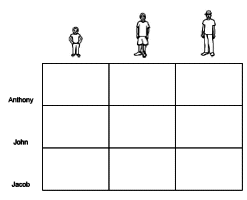Height logic puzzle

Measurement

Have a suggestion or would like to leave feedback?